# Time Domain Analysis of Control System

In a control system, there may be some energy storing elements attached to it. Energy storing elements are generally inductors and capacitors in case of an electrical system. Due to the presence of these energy storing elements, if the energy state of the system is disturbed, it will take a certain time to change from one energy state to another. The exact time taken by the system for changing one energy state to another is known as transient time and the value and pattern voltages and currents during this period are known as the transient response.

A transient response is normally associated with an oscillation, which may be sustained or decaying in nature. The exact nature of the system depends upon the parameters of the system. Any system can be represented with a linear differential equation. The solution of this linear differential equation gives the response of the system. The representation of a control system by a linear differential equation of functions of time and its solution is collectively called time domain analysis of the control system.

### Step Function

Let us take an independent voltage source or a battery which is connected across a voltmeter via a switch, s. It is clear from the figure below, whenever the switch s is open, the voltage appears between the voltmeter terminals is zero. If the voltage between the voltmeter terminals is represented as v (t), the situation can be mathematically represented as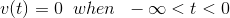Now let us consider at t = 0, the switch is closed and instantly the battery voltage V volt appears across the voltmeter and that situation can be represented as,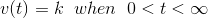Combining the above two equations we get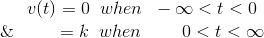In the above equations if we put 1 in place of V, we will get a unit step function which can be defined as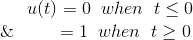Now let us examine the Laplace transform of unit step function. Laplace transform of any function can be obtained by multiplying this function by e-st and integrating multiplied from 0 to infinity.
Fig 6.2.1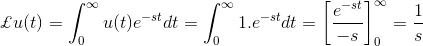If input is R(s), then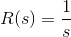### Ramp Function

The function which is represented by an inclined straight line intersecting the origin is known as ramp function. That means this function starts from zero and increases or decreases linearly with time. A ramp function can be represented as,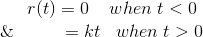Here in this above equation, k is the slope of the line.
Fig 6.2.2
Now let us examine the Laplace transform of ramp function. As we told earlier Laplace transform of any function can be obtained by multiplying this function by e-st and integrating multiplied from 0 to infinity.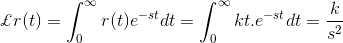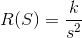### Parabolic Function

Here, the value of function is zero when time t<0 and is quadratic when time t > 0. A parabolic function can be defined as,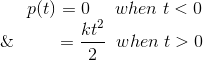Now let us examine the Laplace transform of parabolic function. As we told earlier Laplace transform of any function can be obtained by multiplying this function by e-st and integrating multiplied from 0 to infinity.
Fig 6.2.3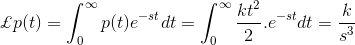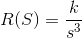## Impulse Function

Impulse signal is produced when input is suddenly applied to the system for infinitesimal duration of time. The waveform of such signal is represented as impulse function. If the magnitude of such function is unity, then the function is called unit impulse function. The first time derivative of step function is impulse function. Hence Laplace transform of unit impulse function is nothing but Laplace transform of first-time derivative of unit step function.
Fig 6.2.4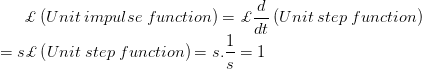### Time Response of First Order Control Systems

When the maximum power of s in the denominator of a transfer function is one, the transfer function represents a first order control system. Commonly, the first order control system can be represented as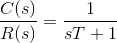#### Time Response for Step Function

Now a unit step input is given to the system, then let us analyze the expression of the output: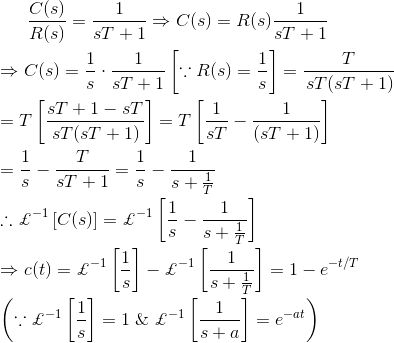Fig 6.3.2It is seen from the error equation that if the time approaching to infinity, the output signal reaches exponentially to the steady-state value of one unit. As the output is approaching towards input exponentially, the steady-state error is zero when time approaches to infinity.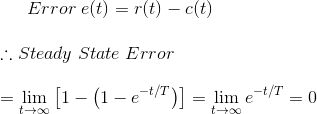Let us put t = T in the output equation and then we get,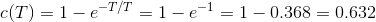This T is defined as the time constant of the response and the time constant of a response signal is that time for which the signal reaches to its 63.2 % of its final value. Now if we put t = 4T in the above output response equation, then we get,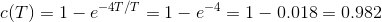When the actual value of the response reaches to the 98% of the desired value, then the signal is said to be reached to its steady-state condition. This required time for reaching the signal to 98 % of its desired value is known as setting time and naturally setting time is four times of the time constant of the response. The condition of response before setting time is known as transient condition and condition of the response after setting time is known as steady-state condition. From this explanation, it is clear that if the time constant of the system is smaller, the response of the system reaches its steady-state condition faster.

#### Time Response for Ramp Function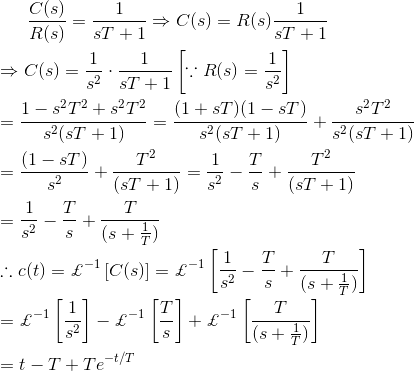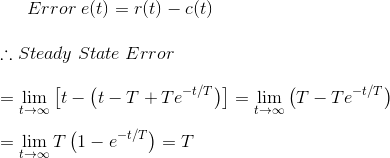In this case, during the steady-state condition, the output signal lags behind the input signal by a time equal to the time constant of the system. If the time constant of the system is smaller, the positional error of the response becomes lesser.

#### Time Response for Impulse Function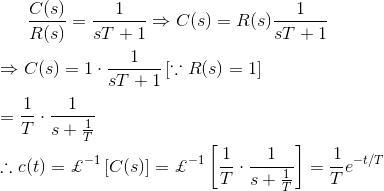In the above explanation of time response of the control system, we have seen that the step function is the first derivative of ramp function and the impulse function is the first derivative of a step function. It is also found that the time response of step function is the first derivative of time response of ramp function and time response of impulse function is the first derivative of time response of step function.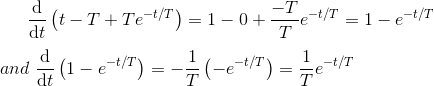Want To Learn Faster? 🎓
Get electrical articles delivered to your inbox every week.
No credit card required—it’s 100% free.